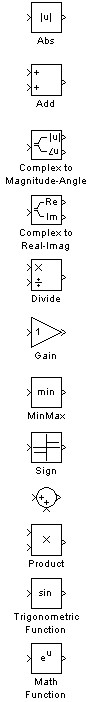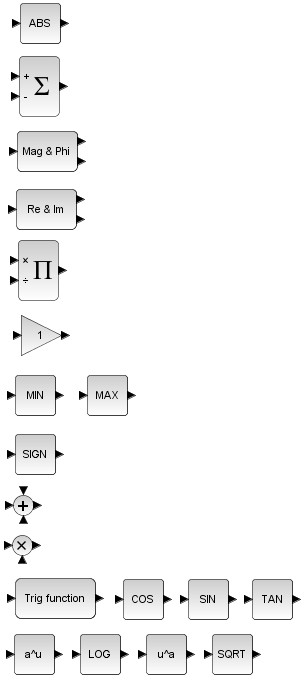# Xcos vs. Simulink® – Mathematical operations library conversion

In the table below you can find the equivalent between the Simulink® and Xcos blocks for Mathematical operations library/palette. The block which are not present in both libraries are omitted from the table.

This conversion table can be used in case you need to convert a Simulink® model to an Xcos block diagram model or vice-versa.

 Simulink Math Operations library Xcos Mathematical Operations paletteImage: Simulink Math Operations tableImage: Xcos Mathematical Operations table

Let’s look at a simple example in which we create a Simulink® block diagram model, simulate it and plot the results. We’ll recreate the equivalent model in Xcos and check if the results are the same.

The purpose of the model is only to use some of the Math Operations Simulink® blocks which have an equivalent in the Xcos palette. The same parameters are going to be used for the Xcos block diagram.

By running the Simulink® model above, we get the following plot window:

### Xcos block diagram model

We follow the same approach for the Xcos block diagram model. Using equivalent blocks (see table above), we recreate the same functionality of the Simulink model.

The block parameters have the same values as those in the Simulink block diagram.

After running the Xcos model for `10 s`, we get the following graphical window:

As you can see, the outputs of the Xcos block diagram model are identical with the Simulink results. This proves that we can use Xcos as an alternative to Simulink, when we want to simulate and analyze block diagrams with mathematical operations.

For any questions, observations and queries regarding this article, use the comment form below.

Don’t forget to Like, Share and Subscribe!